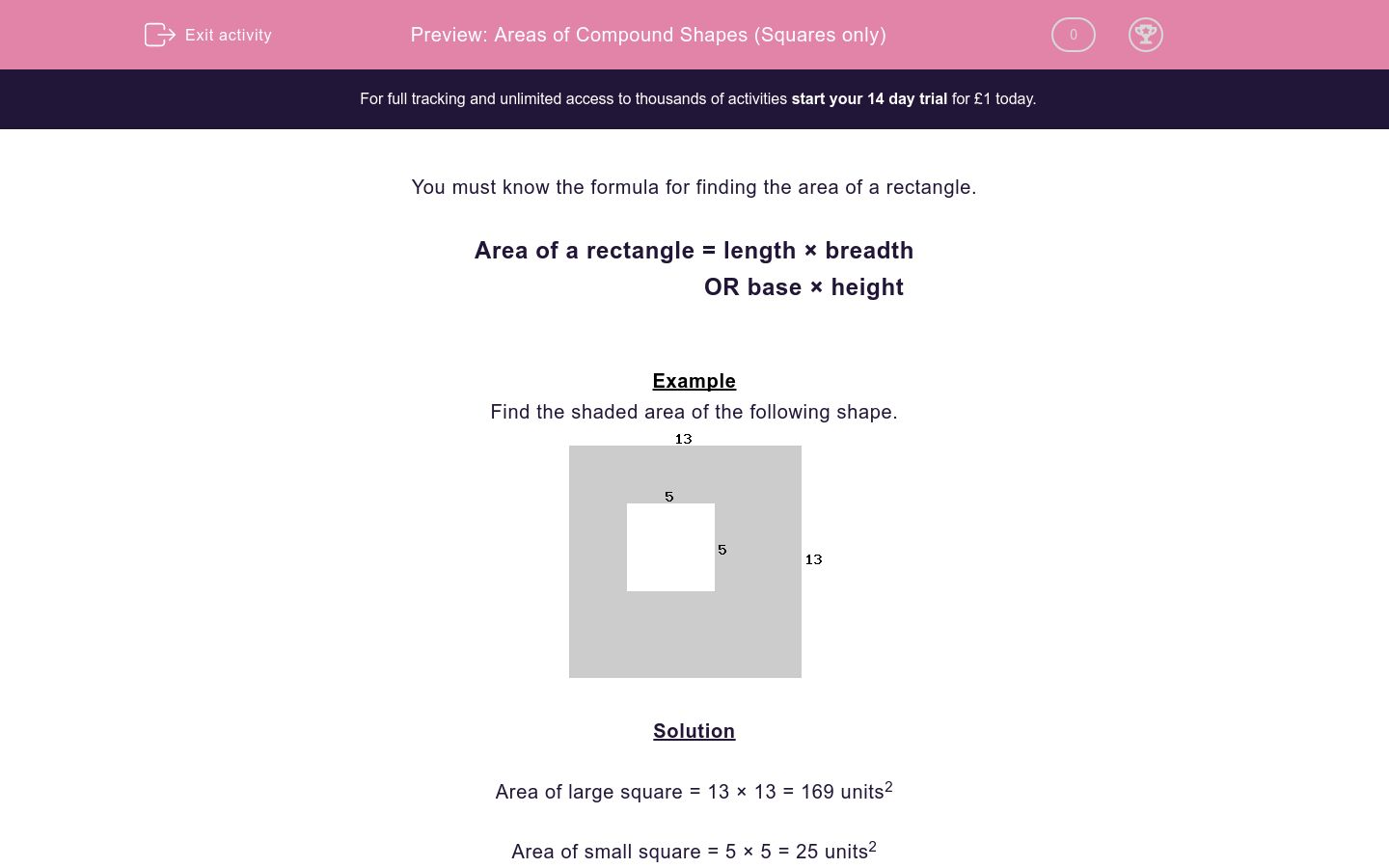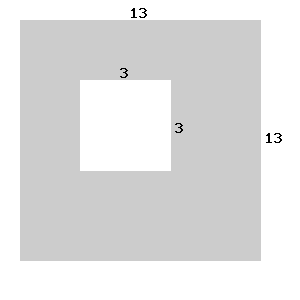# Areas of Compound Shapes (Squares only)

In this worksheet, students find areas of compound shapes made from squares.Key stage:  KS 2

Curriculum topic:   Maths and Numerical Reasoning

Curriculum subtopic:   3D Shapes

Difficulty level:### QUESTION 1 of 10

You must know the formula for finding the area of a rectangle.

Area of a rectangle = length × breadth

OR base × height

Example

Find the shaded area of the following shape.Solution

Area of large square = 13 × 13 = 169 units2

Area of small square = 5 × 5 = 25 units2

Shaded area = 169 − 25 = 144 units2

Find the shaded area of the following shape in cm2. (just write the number)

All of the measurements are given in cm.Find the shaded area of the following shape in cm2. (just write the number)

All of the measurements are given in cm.Find the shaded area of the following shape in cm2. (just write the number)

All of the measurements are given in cm.Find the shaded area of the following shape in cm2. (just write the number)

All of the measurements are given in cm.Find the shaded area of the following shape in cm2. (just write the number)

All of the measurements are given in cm.Find the shaded area of the following shape in cm2. (just write the number)

All of the measurements are given in cm.Find the shaded area of the following shape in cm2. (just write the number)

All of the measurements are given in cm.Find the shaded area of the following shape in cm2. (just write the number)

All of the measurements are given in cm.Find the shaded area of the following shape in cm2. (just write the number)

All of the measurements are given in cm.Find the shaded area of the following shape in cm2. (just write the number)

All of the measurements are given in cm.• Question 1

Find the shaded area of the following shape in cm2. (just write the number)

All of the measurements are given in cm.180
EDDIE SAYS
Area of the large square = 14 x 14 = 196cm2 Area of the small square = 4 x 4 = 16cm2 196 - 16 = 180 cm2
• Question 2

Find the shaded area of the following shape in cm2. (just write the number)

All of the measurements are given in cm.285
EDDIE SAYS
Area of the large square = 17 x 17 = 289cm2 Area of the small square =2 x 2 = 4cm2 289 - 4 = 285 cm2
• Question 3

Find the shaded area of the following shape in cm2. (just write the number)

All of the measurements are given in cm.336
EDDIE SAYS
Area of the large square = 19 x 19 = 361cm2 Area of the small square = 5 x 5 = 25cm2 361 - 25 = 336 cm2
• Question 4

Find the shaded area of the following shape in cm2. (just write the number)

All of the measurements are given in cm.187
EDDIE SAYS
Area of the large square = 14 x 14 = 196cm2 Area of the small square = 3 x 3 = 9cm2 196 - 9 = 187 cm2
• Question 5

Find the shaded area of the following shape in cm2. (just write the number)

All of the measurements are given in cm.200
EDDIE SAYS
Area of the large square = 15 x 15 = 225cm2 Area of the small square =5 x 5 = 25cm2 225 - 25 = 180 cm2
• Question 6

Find the shaded area of the following shape in cm2. (just write the number)

All of the measurements are given in cm.240
EDDIE SAYS
Area of the large square = 16 x 16 = 256 cm2 Area of the small square = 4 x 4 = 16 cm2 256 - 16 = 240 cm2
• Question 7

Find the shaded area of the following shape in cm2. (just write the number)

All of the measurements are given in cm.160
EDDIE SAYS
Area of the large square = 13 x 13 = 169 cm2 Area of the small square = 3 x 3 = 9 cm2 169 - 9 = 160 cm2
• Question 8

Find the shaded area of the following shape in cm2. (just write the number)

All of the measurements are given in cm.391
EDDIE SAYS
Area of the large square = 20 x 20 = 400 cm2 Area of the small square = 3 x 3 = 9 cm2 400 - 9 = 381 cm2
• Question 9

Find the shaded area of the following shape in cm2. (just write the number)

All of the measurements are given in cm.135
EDDIE SAYS
Area of the large square = 12 x 12 = 144cm2 Area of the small square = 3 x 3 = 9cm2 144 - 9 = 135 cm2
• Question 10

Find the shaded area of the following shape in cm2. (just write the number)

All of the measurements are given in cm.144
EDDIE SAYS
Area of the large square = 13 x 13 = 169 cm2 Area of the small square = 5 x 5 = 25 cm2 169 - 25 = 144 cm2
---- OR ----

Sign up for a £1 trial so you can track and measure your child's progress on this activity.

### What is EdPlace?

We're your National Curriculum aligned online education content provider helping each child succeed in English, maths and science from year 1 to GCSE. With an EdPlace account you’ll be able to track and measure progress, helping each child achieve their best. We build confidence and attainment by personalising each child’s learning at a level that suits them.

Get started